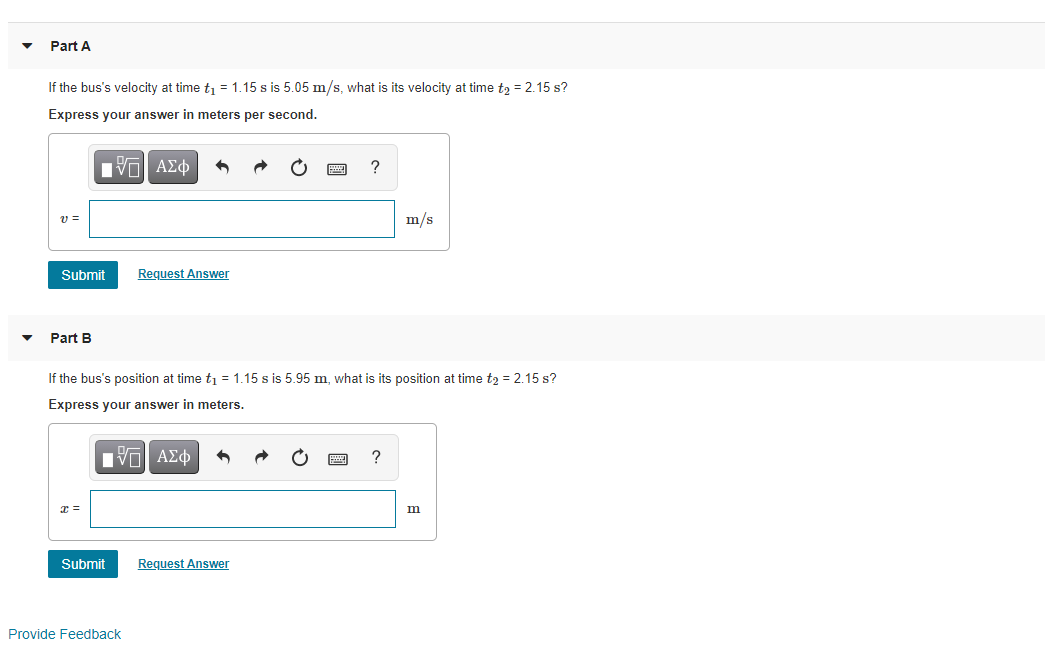# Question Solved1 AnswerQuestion : The acceleration of a bus is givenby ax(t)=αtax(t)=αt, where ααalpha =1.30 m/s3m/s3 is a constant. Part A If the bus's velocity at time t1 = 1.15 s is 5.05 m/s, what is its velocity at time t2 = 2.15 s? Express your answer in meters per second. ΠΙ ΑΣΦ ? V= m/s Submit Request Answer Part B If the bus's position at time ti = 1.15 s is 5.95 m, what is its position at time t2 = 2.15 s? Express your answer in meters. EVO AED m Submit Request Answer Provide FeedbackQuestion : The acceleration of a bus is given by ax(t)=αtax(t)=αt, where ααalpha = 1.30 m/s3m/s3 is a constant.Transcribed Image Text: Part A If the bus's velocity at time t1 = 1.15 s is 5.05 m/s, what is its velocity at time t2 = 2.15 s? Express your answer in meters per second. ΠΙ ΑΣΦ ? V= m/s Submit Request Answer Part B If the bus's position at time ti = 1.15 s is 5.95 m, what is its position at time t2 = 2.15 s? Express your answer in meters. EVO AED m Submit Request Answer Provide Feedback
More
Transcribed Image Text: Part A If the bus's velocity at time t1 = 1.15 s is 5.05 m/s, what is its velocity at time t2 = 2.15 s? Express your answer in meters per second. ΠΙ ΑΣΦ ? V= m/s Submit Request Answer Part B If the bus's position at time ti = 1.15 s is 5.95 m, what is its position at time t2 = 2.15 s? Express your answer in meters. EVO AED m Submit Request Answer Provide Feedback